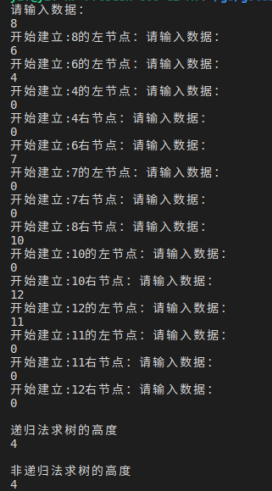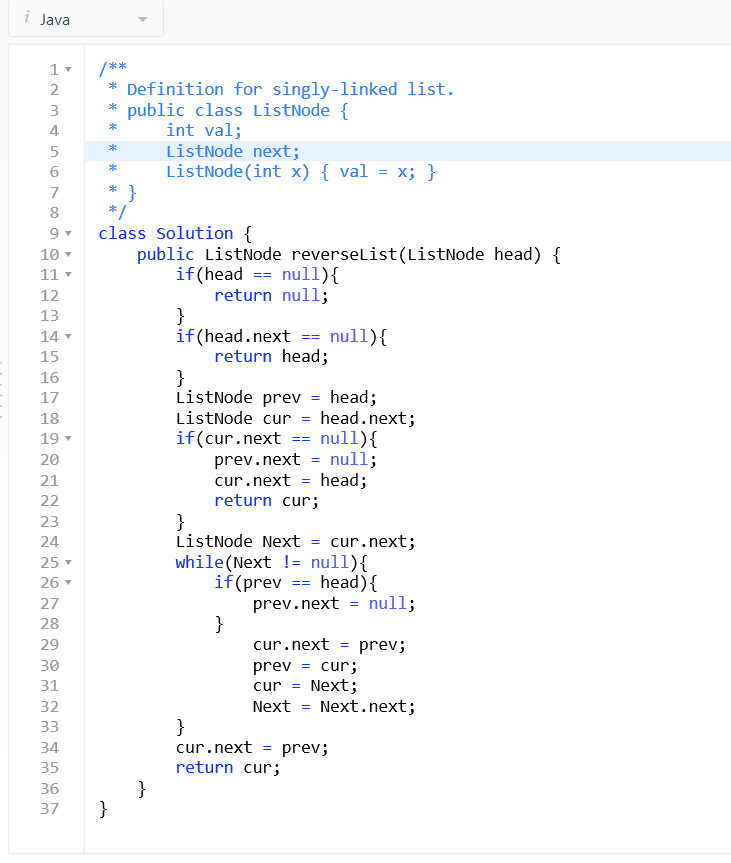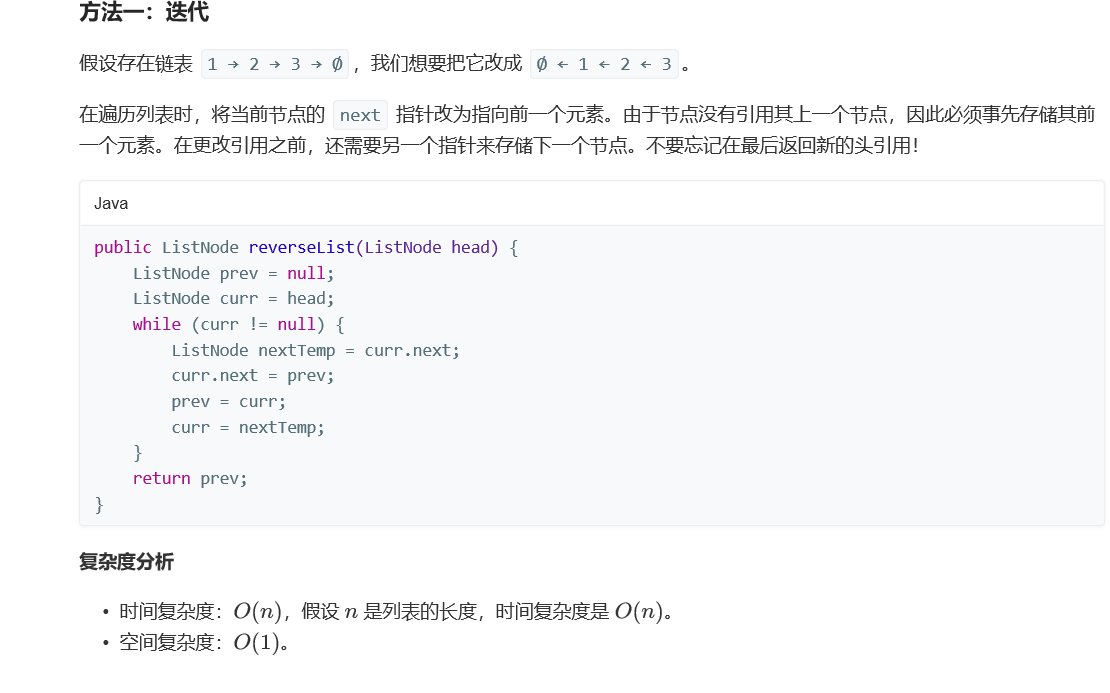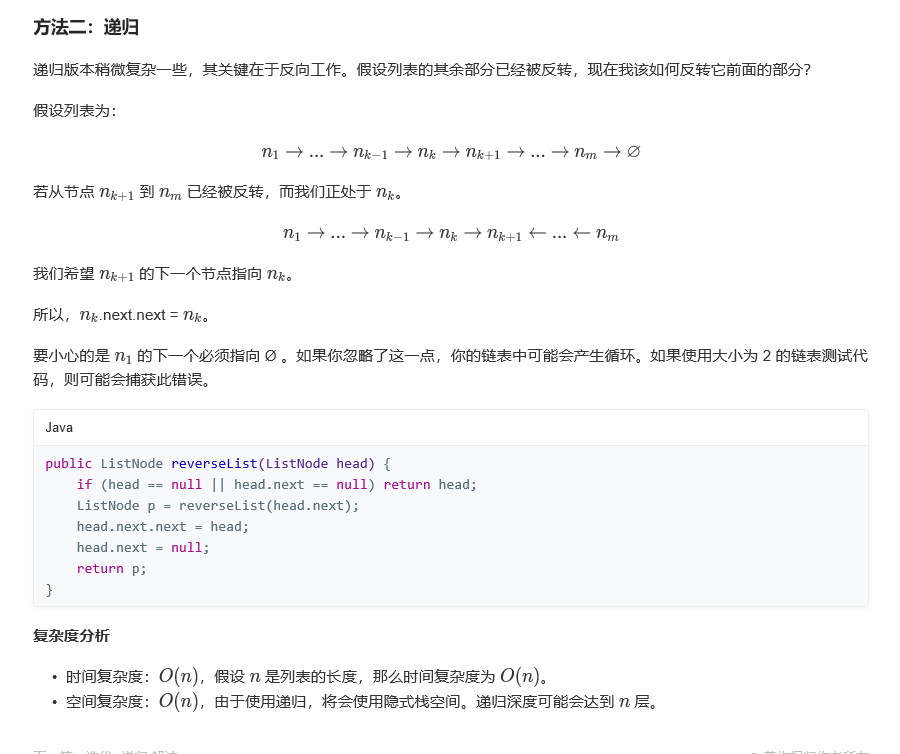•c++
• 递归法实现：用递归法计算Fibonacci（斐波拉契）数列的第n项。C语言
• 主要介绍了C语言之整数划分问题（递归法）实例代码的相关资料,需要的朋友可以参考下
• 递归法求众数，c++实现时间复杂度小于O(n),dev-c,Clion,VScode均编译通过
• 易语言递归法取排列组合例程源码,递归法取排列组合例程,子程序_取组合
• 此代码展示了一种用递归解决迷宫问题的方法，可以自行输入迷宫即得到解答
• C#递归法通用树形菜单，亲测可以用，我这的版本是VS2010和VS2017，不同版本可能会存某些区别，请注意版本。C# 树菜单
• Java 采取递归方法求5!的阶乘，递归方法求阶乘之和，输入要阶乘的数字，递归公式：fn=fn_1*4! 具体来看以下代码： 　System.out.print("输入要阶乘的数字:"); 　Scanner scanner = new Scanner(System.in); 　int n ...
• 递归法 前面我们学习了自定义函数知道函数是为了实现某种功能而编写的一段相对独立的程序并且可以多次的调用 算法描述 function what ( student ) 如果我知道答案那么我就告诉你 否则我要问下一位同学再告诉你 end ...
• 这个是用递归法来写最大公约数，当然原算法还是欧几里得算法；只不过代码比较简洁
• 主要介绍了java递归法求字符串逆序,涉及java递归调用的相关操作技巧,需要的朋友可以参考下
• 算法设计与优化中的递归法德5道经典例题（如最大值，母牛繁殖，x的n次幂等），用c编写，很适合初学者借鉴。
• 主要介绍了Python实现链表反转的方法,结合实例形式分析了Python迭代法与递归法实现链表反转的相关操作技巧与注意事项,需要的朋友可以参考下
• 递归法思路： 树的高度即节点子树的高度+1（节点子树的高度即左子树高度，右子树高度的最大值） 代码如下： // Height_Recursive 递归法求树的高度 int Height_Recursive(TreeNode* pTree) { if (pTree == NULL...
递归法思路：
树的高度即节点子树的高度+1（节点子树的高度即左子树高度，右子树高度的最大值）
代码如下：
// Height_Recursive 递归法求树的高度
int Height_Recursive(TreeNode* pTree) {
if (pTree == NULL)
{
return 0;
}
// 分别求出左子树,右子树的高度,取最大值+1
int leftHeight = Height_Recursive(pTree->left);
int rightHeight = Height_Recursive(pTree->right);
return (leftHeight > rightHeight ? leftHeight: rightHeight)+1;
}
非递归法思路：
借鉴程序遍历的思想，一层层的遍历，累加层数即可得到树的高度
代码如下：
// Height_No_Recursive 非递归法求树的高度
// 思路:借鉴层序遍历的思想,一层层的遍历,层数即高度
int Height_No_Recursive(TreeNode* pTree) {
if (pTree == NULL)
{
return 0;
}
queue<TreeNode*> que;
que.push(pTree);
int height = 0;
TreeNode* p = NULL;
while(!que.empty())
{
height++;
// 该层的节点个数,压入所有节点的孩子节点
int num = que.size();
for (int i=0;i<num;i++)
{
p = que.front();
que.pop();

if (p->left != NULL)
{
que.push(p->left);
}
if (p->right != NULL)
{
que.push(p->right);
}
}
}
return height;
}
实验结果如下图：完整代码如下：
#include<iostream>
#include<queue>
using namespace std;

// 树(节点)定义
struct TreeNode
{
int		    data; // 值
TreeNode*	left; // 左节点
TreeNode*	right;// 右节点
};

// 按照前序建立二叉树，这里我们建立下面的树
//			8
//		6		10
//	  4	  7			12
//                11
// 所以输入顺序是：8 6 4 0 0 7 0 0 10 0 12 11 0 0 0
void createTree(TreeNode*& t)
{
cout<<"请输入数据："<<endl;
int val = 0;
cin>>val;

// 0表结束
if (0 == val)
{
t = NULL;
}
else
{
t = new TreeNode();
t->data = val;
cout<<"开始建立:"<<val<<"的左节点：";
createTree(t->left);
cout<<"开始建立:"<<val<<"右节点：";
createTree(t->right);
}
}

// Height_Recursive 递归法求树的高度
int Height_Recursive(TreeNode* pTree) {
if (pTree == NULL)
{
return 0;
}
// 分别求出左子树,右子树的高度,取最大值+1
int leftHeight = Height_Recursive(pTree->left);
int rightHeight = Height_Recursive(pTree->right);
return (leftHeight > rightHeight ? leftHeight: rightHeight)+1;
}

// Height_No_Recursive 非递归法求树的高度
// 思路:借鉴层序遍历的思想,一层层的遍历,层数即高度
int Height_No_Recursive(TreeNode* pTree) {
if (pTree == NULL)
{
return 0;
}
queue<TreeNode*> que;
que.push(pTree);
int height = 0;
TreeNode* p = NULL;
while(!que.empty())
{
height++;
// 该层的节点个数,压入所有节点的孩子节点
int num = que.size();
for (int i=0;i<num;i++)
{
p = que.front();
que.pop();

if (p->left != NULL)
{
que.push(p->left);
}
if (p->right != NULL)
{
que.push(p->right);
}
}
}
return height;
}

int main()
{
TreeNode* pTree = NULL;
createTree(pTree);

cout<<"\n递归法求树的高度"<<endl;
cout<<Height_Recursive(pTree)<<endl;

cout<<"\n非递归法求树的高度"<<endl;
cout<<Height_No_Recursive(pTree)<<endl;

return 0;
}

展开全文树的高度 二叉树高度
• 易语言递归法取排列组合例程（M选N递归法），源码可以学习到递归法易语言例程
• 主要介绍了Python 实现递归法解决迷宫问题的示例代码，文中通过示例代码介绍的非常详细，对大家的学习或者工作具有一定的参考学习价值，需要的朋友们下面随着小编来一起学习学习吧
• 今天整理了一下用递归法求最大公约数(gcd)和最小公倍数(lcm)。主要的工作是求最大公约数。数学上可以用辗转法求最大公约数
• 内容索引:C#源码,菜单窗体,树状菜单,递归法　用C#递归法实现的通用树状菜单，菜单数据来自数据库，运行于VS2005，编译通过，你可把它改进为你软件的一个模块，方便以后使用。
• 主要为大家详细介绍了python递归法解决棋盘分割问题，具有一定的参考价值，感兴趣的小伙伴们可以参考一下
• 递归法取排列组合易语言源码例程.rar 递归法取排列组合易语言源码例程.rar 递归法取排列组合易语言源码例程.rar 递归法取排列组合易语言源码例程.rar 递归法取排列组合易语言源码例程.rar 递归法取排列组合...
• 易语言源码递归法取排列组合易语言源码例程.rar 易语言源码递归法取排列组合易语言源码例程.rar 易语言源码递归法取排列组合易语言源码例程.rar 易语言源码递归法取排列组合易语言源码例程.rar 易语言源码递归法...
• 主要介绍了php递归法读取目录及文件的方法,是非常经典的递归算法,涉及php操作文件及目录的技巧,需要的朋友可以参考下
• C语言 递归法求阶乘 利用一个函数进行递归求阶乘，但该数不能小于0，且若为0或1，得到的结果都为1，其他情况，对该数不断递归 #include <stdio.h> #include <stdlib.h> int main() { int fac(int n); /...
C语言 递归法求阶乘
利用一个函数进行递归求阶乘，但该数不能小于0，且若为0或1，得到的结果都为1，其他情况，对该数不断递归
#include <stdio.h>
#include <stdlib.h>
int main()
{
int fac(int n);              // 函数声明
int n;             //声明要阶乘的数为int型
int y;             //声明结果为int型
scanf("%d",&n);              // 输入要阶乘的数
y=fac(n);
printf("%d!=%d\n",n,y);
system("pause");
return 0;
}

int fac(int n)                // 定义fac函数
{
int f;
if(n<0)                     // n不能小于0
printf("n<0,data error!");
else if( n==0 || n==1)         // n=0 or n=1 ，阶乘都为1
f=1;
else f=fac(n-1)*n;           // n>1时，n!=n*(n-1)
return(f);
}
//递归法求阶乘

展开全文c语言
• 最近在leetcode看到一道反转单链表的题，题目难度并不大，但是提交成功之后，看了官方给的递归版本的答案，觉得有点意思。以下是题目和代码，和大家分享一下 ...以下是官方给的迭代以及递归的标准答案 ...
最近在leetcode看到一道反转单链表的题，题目难度并不大，但是提交成功之后，看了官方给的递归版本的答案，觉得有点意思。以下是题目和代码，和大家分享一下下图是题目模板以及我自己写的代码（迭代法，和官方给的比起来就有点捞了）以下是官方给的迭代法以及递归的标准答案关于递归版本的答案，我再此陈述一下自己的理解。 这一部分是递归跳出的条件，跳出时说明已到尾节点
if(head == null||head.next == null){
}

这一部分只是记录反转后的头结点及反准之前的尾节点。
 Node p = reverseList(head.next);

 head.next.next = head;

展开全文• 主要介绍了Java求解两个非负整数最大公约数算法,结合实例形式分析了java求解最大公约数的实现方法,并附带了循环法与递归法算法思路,需要的朋友可以参考下
• 递推法 #include<stdio.h> int main() { int n,y=1,i; scanf("%d",&n); if(n==0||n==1) y=1;//n是0或1的时候阶乘都是1 else for( i=1;...递归法 //函数的递归使用 #include<stdi
递推法
#include<stdio.h>
int main()
{
int n,y=1,i;
scanf("%d",&n);
if(n==0||n==1) y=1;//n是0或1的时候阶乘都是1
else for( i=1;i<=n;i++)//从1开始乘，一直乘到n
{
y=i*y;//每次让i乘他前面数的阶乘
}
printf("%d!=%d",n,y);
return 0;
}

递归法
//函数的递归使用
#include<stdio.h>
int ff(int n)
{
long f;
if(n<0) printf("n<0,input error");
else if(n==0||n==1) f=1;
else f=ff(n-1)*n;
return (f);
}
int main()
{
int n;
long y;
printf("input a int eager number:\n");
scanf("%d",&n);
y=ff(n);
printf("%d!=%ld",n,y);
return 0;

}

展开全文c语言
• 利用递归方法求5!，递归公式：fn=fn_1*4!
• //递归法分解质因数// #include"feng.h" int fenjiezhiyinshu(long long int large); int main () { long long large; printf("Large integer="); scanf("%lld",&large); fenjiezhiyinshu(large); puts(...c语言 算法...